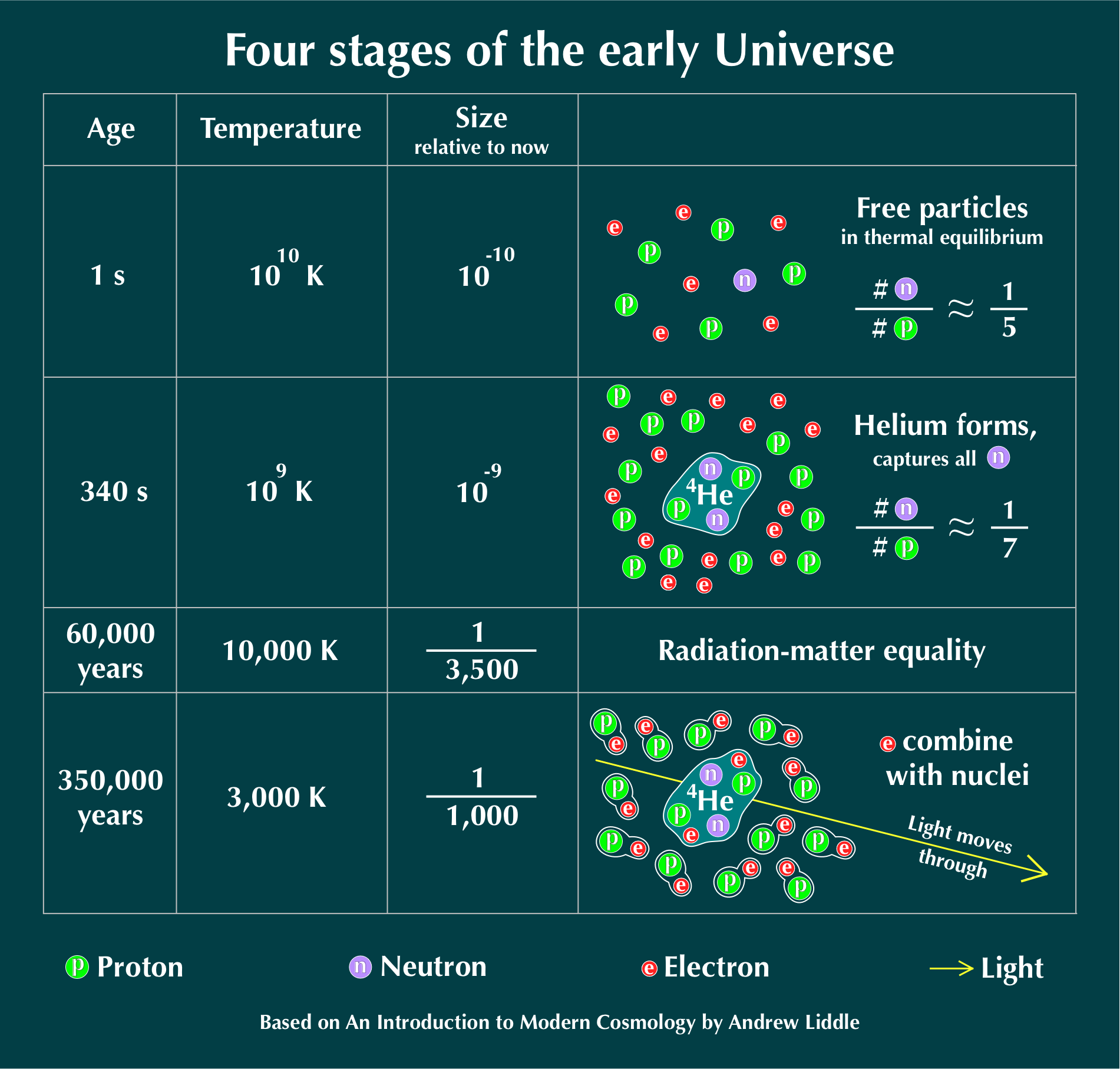# Four stages of the early Universe

The following image shows four stages of evolution of the early Universe, from 1 second to 350 thousand years since the Big Bang. During that period the Universe cooled as it expanded in size. This article is based on excellent textbook An Introduction to Modern Cosmology by Andrew Liddle.Figure 1: Four stages of the Early Universe.

## Universe at one second after the Big Bang

At one second after the Big band the Universe was a hot soup of freely moving particles - protons, neutrons and electrons. In these high energy conditions the protons and neutrons were transforming into each other. The two particles have slightly different masses, and this mass difference determined the neutron-to-proton ratio: for each neutron there were five protons.

## Universe at 340 seconds

At 340 seconds, the Universe cools down enough for complex nuclei like Helium to exist. The Helium-4 is made of two protons and two neutrons, and at these temperatures most particles are no longer moving fast enough to break Helium down into free particles. The formation of Helium consumes all existing neutrons, resulting in the Universe consisting mostly of single protons (also called Hydrogen nuclei), Helium-4 nuclei and free electrons. During the period between 1 and 340 seconds some neutrons decay into protons, which decreases the neutron-to-proton ratio from 1/5 to 1/7. This ratio remains fixed to the present day and can be observed.

### Helium abundance is 25%

Another very important quantity that is fixed at 340 seconds is the abundance of Helium-4 nuclei in the Universe. This abundance is observed in the old globular clusters to be about 25%, which means that 25% of the the total mass of all the particles in the Universe are in the form of Helium-4. Here is how this abundance can be found theoretically.

Firstly, we use the fact that a Helium-4 nucleus contains two neutrons and two protons. Secondly, we assume that after 340 seconds there is one neutron per seven protons in the Universe. Therefore, for each Helium nucleus there are also 12 free protons (Figure 2).Figure 2: Calculating the number of helium nuclei and free protons in the Universe, which is 340 seconds old, given the neutron-to-proton ratio of 1/7.

The abundance of Helium-4 is the mass of Helium-4 nuclei divided by the mass of all particles (including Helium-4). If we assume that protons and neutrons have the same mass, this ratio turns out to be precisely 1/4 (Figure 3).Figure 3: Calculating the primordial helium abundance.

This means that after 360 seconds, the Universe consisted of 25% Helium-4 and 75% Hydrogen (neglecting very small amounts of other elements). This is the starting chemical composition of the Universe before formation of stars and other large objects, and this composition is measured to be mostly unchanged to the present day.

## Universe at 60,000 years

The Universe becomes less dense as it expands. The density of both particles and light decreases. However, it turns out that density of light decreases faster than the density of particles as the Universe becomes larger. As a result, the density of matter becomes equal to the density of light at some time during expansion. Cosmological models predict that this happens when the Universe is about 60,000 years old. Since then, there is more matter per cubic meter than light.

## Universe at 350,000 years and the cosmic microwave background

Another very important transition happens when the Universe is about 350,000 years old. Before that time, Helium and Hydrogen nuclei did not have electrons because it was too hot for the electrons to remain attached to the nuclei. An electron that became bound to an nucleus was immediately knocked off by an energetic photon. As Universe expanded, the photons became less energetic, and at 350,000 years most photons could no longer prevent electrons form binding to Helium and Hydrogen. As a result, the nuclei captured electrons, and light was able to move freely through space without bumping into free electrons. In other words, the Universe became transparent.

Some photons that were emitted when the Universe was 350,000 years old are still traveling through space today and are being detected by astronomers. This old light is called cosmic microwave background.

• An Introduction to Modern Cosmology 3rd Edition by Andrew Liddle Publisher, Amazon.